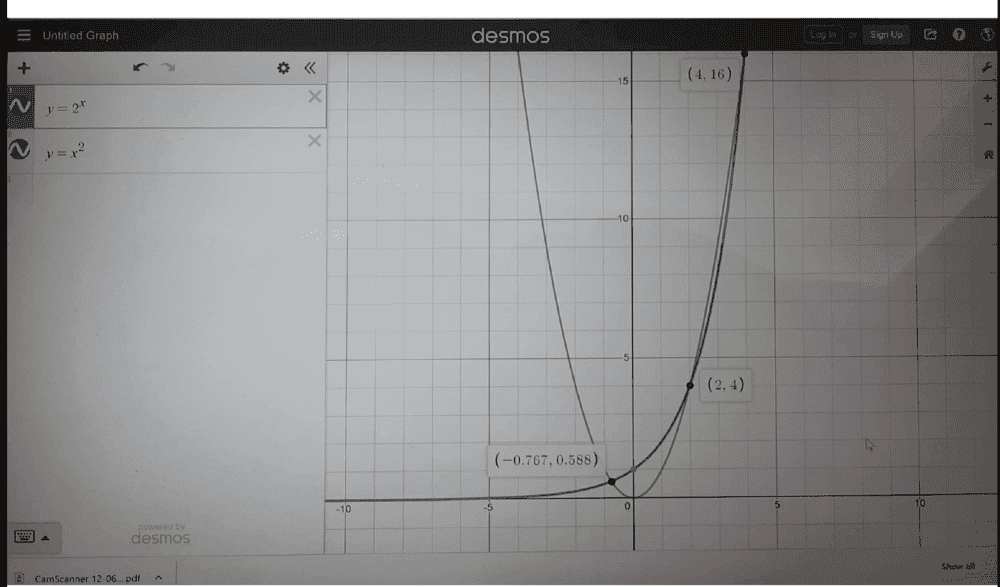# Solve for x in this index notation problem

Gold Member
Homework Statement:
##2^x##=##x^2##
Relevant Equations:
trial/error method
ok, by direct substitution i know that either ##x=2## or ##x=4##
but i would like to prove this analytically, would it be correct saying,
##xln 2= 2ln x##
##xln_{2}2=2 ln_{2}x##
##x=2 ln_{2}x##
##\frac {1}{2}=\frac { ln_{2}x}{x}##
##ln_{2}x^{1/x}##=##\frac {1}{2}##
→##2^{1/2}##=##x^{1/x}##
→##x=2##
is my thinking correct? and how do we show the solution ##x=4?## analytically

Gold Member
I just saw it on another group, where they simply used trial and error to get ##x=2## and ##x=4##

Gold Member
I doubt you can solve this analytically. Of course, you have made two guesses, but the question is: are there more? The answer turns out to be yes: https://www.wolframalpha.com/input/?i=2^x+=+x^2

Where did you find this question?
I have made guesses yes and at the same time I have tried to solve the problem and I wanted a feedback on that approach sir.

Homework Helper
Gold Member
If one writes ##x^y=y^x## isn't it obvious by symmetry that ##x=y## is always one of the solutions?

Gold Member
If one writes ##x^y=y^x## isn't it obvious by symmetry that ##x=y## is always one of the solutions?

i do not disagree,...true, i just wanted to work from the original problem to realize a solution. It is you to inform me whether my steps make sense or not, i have tried showing that, ...yes by symmetry, we would immediately get the solution to the problem as indicated...

Staff Emeritus
Homework Helper
Homework Statement:: ##2^x##=##x^2##
Relevant Equations:: trial/error method

ok, by direct substitution i know that either ##x=2## or ##x=4##
but i would like to prove this analytically, would it be correct saying,
##xln 2= 2ln x##
You've implicitly assumed ##x>0## here.

##xln_{2}2=2 ln_{2}x##
##x=2 ln_{2}x##
##\frac {1}{2}=\frac { ln_{2}x}{x}##
##ln_{2}x^{1/x}##=##\frac {1}{2}##
→##2^{1/2}##=##x^{1/x}##
→##x=2##
is my thinking correct? and how do we show the solution ##x=4?## analytically
Looks okay, but it doesn't seem like the work really bought you anything. To get to ##x=2## in the last step, you're essentially using the same logic you used to get the solution to the original form, i.e., if you plug in 2, both sides are obviously equal.

The most insightful approach (to me) would be to plot the two functions and see where they intersect. To get the negative root, you'd have to use numerical methods.

•chwala
Gold Member
You've implicitly assumed ##x>0## here.

Looks okay, but it doesn't seem like the work really bought you anything. To get to ##x=2## in the last step, you're essentially using the same logic you used to get the solution to the original form, i.e., if you plug in 2, both sides are obviously equal.

The most insightful approach (to me) would be to plot the two functions and see where they intersect. To get the negative root, you'd have to use numerical methods.
Ok, thanks for your feedback...noted with regards.

Gold Member
You've implicitly assumed ##x>0## here.

Looks okay, but it doesn't seem like the work really bought you anything. To get to ##x=2## in the last step, you're essentially using the same logic you used to get the solution to the original form, i.e., if you plug in 2, both sides are obviously equal.

The most insightful approach (to me) would be to plot the two functions and see where they intersect. To get the negative root, you'd have to use numerical methods.
Which numerical methods would you suggest for the negative roots...

Staff Emeritus
Homework Helper
I like Newton-Raphson.

•chwala
Gold Member
I know it...I will check it out. Cheers

Gold Memberusing the graph we get all the solutions...##x=-0.767##, let me check on how to arrive at this using Newton-raphson method...long since i looked at this numerical methods...cheers

Gold Member
so we need to let ##f(x)= 2^x -x^2## for us to check the roots btwn ##x=-1## and ##x=1## rather than,
##f(x)=x^2-2^x##, or it does not matter?
note that at ##f(-1)= -0.5## and at ##f(1)=1## implying that the roots lie in between.
ok, i am getting, ##f'(x)=2^x ln 2 -2x##
##x_{1}=x_{0}- \frac {f(x_{0})}{f'(x_{0})}##
##x_{1}=-1-\frac{-0.5}{2.3465}##
##x_{1}=-1+0.213=-0.787##
am i on the correct path...

Last edited:
Gold Member
What about the lambert w function?is it a reliable way of solving the same problem...just saw it on YouTube.

Last edited:
Staff Emeritus
Gold Member
I mean... Kind of. But if you asked someone to solve the equation ##xe^x=2## and they told you the answer was W(2), would you consider yourself satisfied? That's the level of satisfaction you'll get using that function. If you ask how to evaluate W, it's going to be the Newton-Rhapsody method or something largely equivalent.

•chwala
Mentor
Newton-Rhapsody
Spell checker? LOL!

Gold Member
😃😃😃 a bit of humour is good during this hard times...

Last edited:
Mayhem
I mean... Kind of. But if you asked someone to solve the equation ##xe^x=2## and they told you the answer was W(2), would you consider yourself satisfied? That's the level of satisfaction you'll get using that function. If you ask how to evaluate W, it's going to be the Newton-Rhapsody method or something largely equivalent.
If you can find peace in ln(2) being a satisfying answer, I would W(2) should be equally satisfactory.

Staff Emeritus